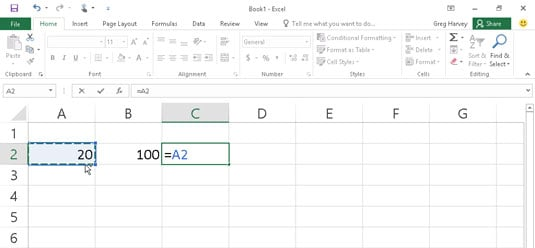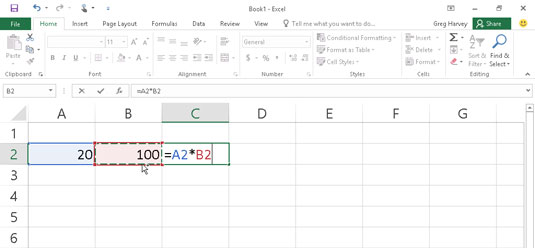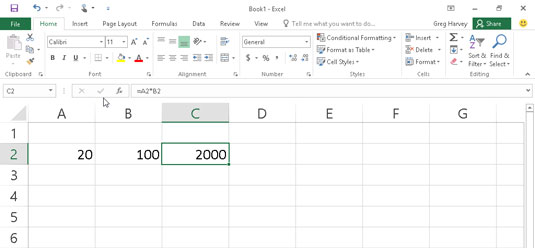##### Excel 2016 For DummiesAs entries go in Excel 2016, formulas are the real workhorses of the worksheet. If you set up a formula properly, it computes the correct answer when you enter the formula into a cell. From then on, the formula stays up to date, recalculating the results whenever you change any of the values that the formula uses.

You let Excel know that you're about to enter a formula (rather than some text or a value) in the current cell by starting the formula with the equal sign (=). Most simple formulas follow the equal sign with a built-in function, such as SUM or AVERAGE. Other simple formulas use a series of values or cell references that contain values separated by one or more of the following mathematical operators:

• + (plus sign) for addition

• – (minus sign or hyphen) for subtraction

• * (asterisk) for multiplication

• / (slash) for division

• ^ (caret) for raising a number to an exponential power

For example, to create a formula in cell C2 that multiplies a value entered in cell A2 by a value in cell B2, enter the following formula in cell C2: =A2*B2.

To enter this formula in cell C2, follow these steps:

1. Select cell C2.

2. Type the entire formula =A2*B2 in the cell.

3. Press Enter.

Or

1. Select cell C2.

2. Type = (equal sign).

3. Select cell A2 in the worksheet by using the mouse or the keyboard.

This action places the cell reference A2 in the formula in the cell (as shown here).To start the formula, type = and then select cell A2.
4. Type * (Shift+8 on the top row of the keyboard).

The asterisk is used for multiplication rather than the x symbol you used in school.

5. Select cell B2 in the worksheet with the mouse, keyboard, or by tapping it on the screen (when using a touchscreen device).

This action places the cell reference B2 in the formula (as shown here).To complete the second part of the formula, type * and select cell B2.
6. Click the Enter button on the Formula bar to complete the formula entry while keeping the cell pointer in cell C2.

Excel displays the calculated answer in cell C2 and the formula =A2*B2 in the Formula bar (as shown here).Click the Enter button, and Excel displays the answer in cell C2 while the formula appears in the Formula bar above.

When you finish entering the formula =A2*B2 in cell C2 of the worksheet, Excel displays the calculated result, depending on the values currently entered in cells A2 and B2. The major strength of the electronic spreadsheet is the capability of formulas to change their calculated results automatically to match changes in the cells referenced by the formulas.

Now comes the fun part: After creating a formula like the preceding one that refers to the values in certain cells (rather than containing those values itself), you can change the values in those cells, and Excel automatically recalculates the formula, using these new values and displaying the updated answer in the worksheet!

Using the example shown, suppose that you change the value in cell B2 from 100 to 50. The moment that you complete this change in cell B2, Excel recalculates the formula and displays the new answer, 1000, in cell C2.